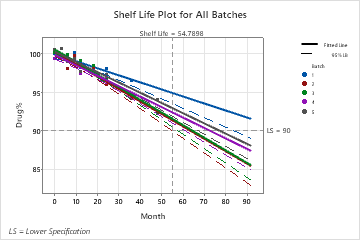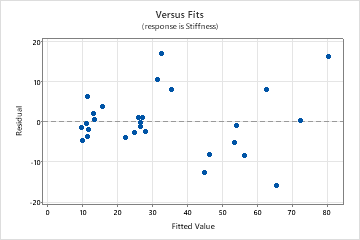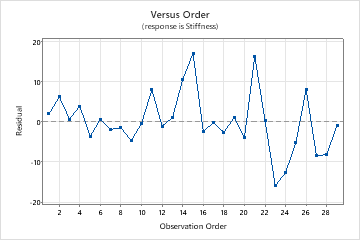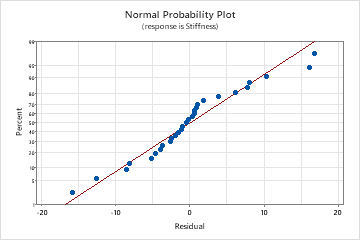# Interpret the key results for Stability Study

Complete the following steps to interpret a stability study. Key output includes the p-value, coefficients, shelf life estimates, regression equations, model summary statistics, and the residual plots.

## Step 1: Determine whether the association between the response and the term is statistically significant

If your data include a batch factor, then the model selection table shows the results of the model selection process. Minitab uses the final model from the selection process to estimate shelf life.

Minitab starts with the full model that includes time, batch, and the batch by time interaction. Minitab then compares the p-value for the interaction to the value specified in Alpha for pooling batches (also called α). If the p-value for the interaction is less than α, then the model cannot be reduced. The final model includes all three terms.

If the p-value for the interaction is greater than or equal to α, then Minitab removes the interaction and evaluates the reduced model with only time and batch. If the p-value for batch in the reduced model is less than α, then the model cannot be reduced further. The final model includes time and batch.

If the p-value for batch in the reduced model is greater than or equal to α, then Minitab removes batch. The final model includes only time.

## Step 2: Determine shelf life of the product

The shelf life estimation table shows the specification limits, the confidence level that is used to calculate the shelf life, and the shelf life estimates.

If the batch factor is fixed and not included in the final model, then the shelf life is the same for all batches. Otherwise, the shelf life for each batch is different, and Minitab displays the shelf life estimate for each batch. The overall shelf life for the product is equal to the smallest of the individual shelf life values.

If the batch factor is random, Minitab only calculates the overall shelf life.Key results: Shelf life estimates, shelf life plot In these results, the final model includes the batch factor, so Minitab displays the shelf life estimates for each batch. The overall shelf life estimate is 54.79 months. This value is the shelf life for Batch 2, which has the shortest shelf life.

## Step 3: Examine how the term is associated with the response

For a fixed batch factor, if time is the only term in the final model, then all batches share the same intercept and slope, and Minitab displays a single regression equation. Otherwise, Minitab displays a separate equation for each batch. If the batch factor is included in the final model, but the batch by time interaction is not included, then all batches have different intercepts but the same rate of degradation. If both the batch term and the batch by time interaction term are included in the final model, then all batches have different intercepts and different slopes.

## Step 4: Determine how well the model fits your data

To determine how well the model fits your data, examine the goodness-of-fit statistics in the Model Summary table.

R-sq

R2 is the percentage of variation in the response that is explained by the model. The higher the R2 value, the better the model fits your data. R2 is always between 0% and 100%.

R2 always increases when you add additional predictors to a model. For example, the best five-predictor model will always have an R2 that is at least as high as the best four-predictor model. Therefore, R2 is most useful when you compare models of the same size.

Use adjusted R2 when you want to compare models that have different numbers of predictors. R2 always increases when you add a predictor to the model, even when there is no real improvement to the model. The adjusted R2 value incorporates the number of predictors in the model to help you choose the correct model.

Consider the following when you compare the R2 values:
• Small samples do not provide a precise estimate of the strength of the relationship between the response and predictors. For example, if you need R2 to be more precise, you should use a larger sample (typically, 40 or more).

• Goodness-of-fit statistics are just one measure of how well the model fits the data. Even when a model has a desirable value, you should check the residual plots to verify that the model meets the model assumptions.

## Step 5: Determine whether your model meets the assumptions of the analysis

Use the residual plots to help you determine whether the model is adequate and meets the assumptions of the analysis. If the assumptions are not met, the model may not fit the data well and you should use caution when you interpret the results.

###### Note

If your model includes a random batch factor, you can plot marginal and conditional residuals. The marginal fits are the fitted values for the overall population. Use the conditional residuals to check the normality of the error term in the model.

### Residuals versus fits plot

Use the residuals versus fits plot to verify the assumption that the residuals are randomly distributed and have constant variance. Ideally, the points should fall randomly on both sides of 0, with no recognizable patterns in the points.

The patterns in the following table may indicate that the model does not meet the model assumptions.
Pattern What the pattern may indicate
Fanning or uneven spreading of residuals across fitted values Nonconstant variance
Curvilinear A missing higher-order term
A point that is far away from zero An outlier
A point that is far away from the other points in the x-direction An influential point
In this residuals versus fits plot, the points appear randomly scattered on the plot. However, the point in the upper right corner appears to be an outlier. Try to identify the cause of the outlier. Correct any data entry or measurement errors. Consider removing data values that are associated with abnormal, one-time events (special causes). Then, repeat the analysis.### Residuals versus order plot

Use the residuals versus order plot to verify the assumption that the residuals are independent from one another. Independent residuals show no trends or patterns when displayed in time order. Patterns in the points may indicate that residuals near each other may be correlated, and thus, not independent. Ideally, the residuals on the plot should fall randomly around the center line:If you see a pattern, investigate the cause. The following types of patterns may indicate that the residuals are dependent.
In this residuals versus order plot, the outlier that is also visible on the other residual plots appears to correspond to the observation in row 21 of the worksheet.### Normal probability plot

Use the normal probability plot of the residuals to verify the assumption that the residuals are normally distributed. The normal probability plot of the residuals should approximately follow a straight line.

The patterns in the following table may indicate that the model does not meet the model assumptions.
Pattern What the pattern may indicate
Not a straight line Nonnormality
A point that is far away from the line An outlier
Changing slope An unidentified variable
In this normal probability plot, the residuals generally appear to follow a straight line. However, the point in the upper right corner of the plot is far away from the line and appears to be an outlier, which was also visible on the other residual plots.For more information on how to handle patterns in the residual plots, go to Residual plots for Fitted Line Plot.

By using this site you agree to the use of cookies for analytics and personalized content.  Read our policy